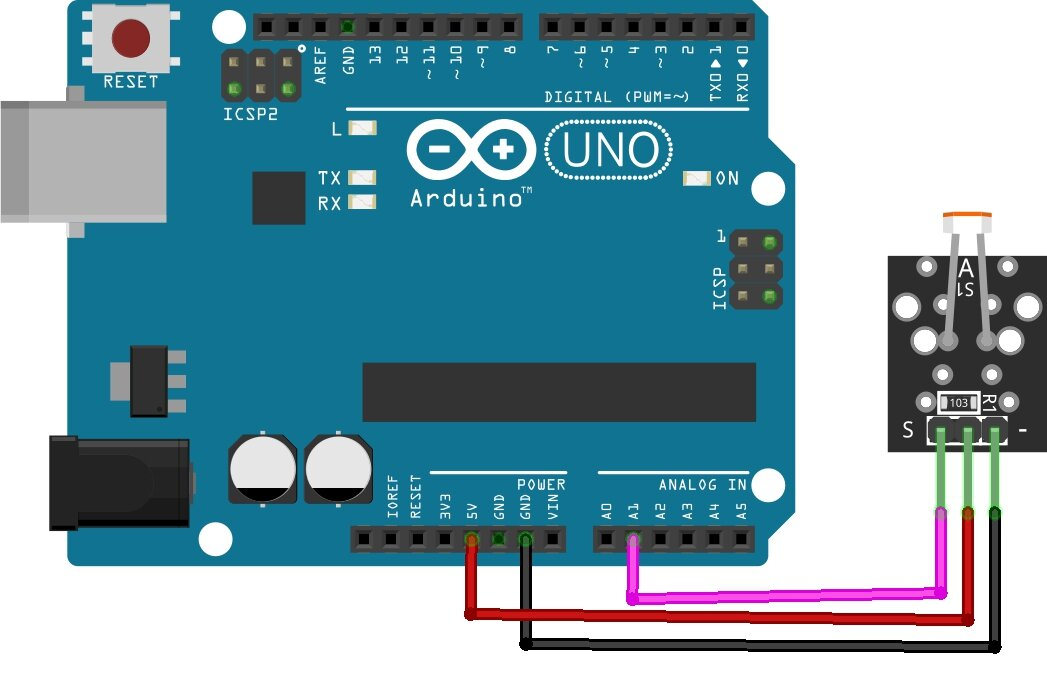Arduino Projects

# How to Use an LDR Sensor With Arduino Tutorial

An LDR sensor is a light-dependent resistor. It is used to detect the intensity of light. LDR Sensor With Arduino The resistance of an LDR sensor decreases with the power of light. The LDR sensor is used in many applications such as light sensing, intensity detection, etc. The LDR sensor can be interfaced with an Arduino using the analogue input pin. The voltage at the analogue input pin is proportional to the intensity of light. The LDR sensor is connected to the analog input pin of the Arduino.

## LDR SensorAn LDR sensor is a type of light-dependent resistor which is used to measure the intensity of light. It is a critical component in many light-sensitive applications such as security systems, cameras, and lighting control. The resistance of an LDR sensor decreases as the intensity of light increases. This makes it an ideal sensor for measuring light intensity. LDR sensors are commonly used in light-sensitive applications such as security systems and lighting control.

### LDR Sensor module pinoutThe LDR sensor module has three pins: power, ground and signal. The power and ground pins are used to power the sensor, while the signal pin is used to output the sensor’s readings. the LDR sensor module is a simple and inexpensive way to measure light intensity. It is a versatile sensor that can be used in a variety of applications.

### Specification:

• Input Voltage: DC 3.3V to 5V VCC
• Output: Analog and Digital
• External GND GND

### Applications

• Detect Day or Night
• Automatic bedroom Lights
• Automatic Rear view mirror
• Automatic Street Light

## Schematic diagramThe ground lead is connected to the ground pin on the Arduino board. The power lead is connected to the +5V pin on the Arduino board. The output lead is connected to the digital input/output (I/O) pin on the Arduino board.

The LDR is connected to the Arduino via the A1 pin.

## Sample Source Code – LDR Sensor With Arduino

#### The basic script for measuring LDR values

```// A0:

// the setup routine runs once when you press reset:
void setup() {
// initialize serial communication at 9600 bits per second:
Serial.begin(9600);
}

// the loop routine runs over and over again forever:
void loop() {
// read the input on analog pin A1:
int sensorValue = analogRead(A1);
// Convert the analog reading (which goes from 0 - 1023) to a voltage (0 - 5V):
float voltage = sensorValue * (5.0 / 1024.0);

// the lower the voltage, the brighter it is
if ((voltage >= 0) && (voltage <= 0.4)) {
Serial.print ("it is light - ");
} else if ((voltage > 0.4) && (voltage <= 2)) {
Serial.print ("it is bright - ");
} else {
Serial.print ("it is dark - ");
}
// print out the value you read:
Serial.println(voltage);

// D0:

// when sensor pin D0 is connected, the sensor only knows the state light (0.14V) and dark (5.0V).
// The brightness at which the particular state is to be set can be set using the rotary potentiometer.

}```
```void setup()
{
Serial.begin(9600);
}
void loop()
{
unsigned int AnalogValue;

Serial.println(AnalogValue);

}```

#### Arduino Code For LDR diagram and lighting control with the relay

```int  LedPin  =  10 ;  // Pin to turn on the led
int  sensorPin  =  A5 ;  // Selection of LDR input pin
int  sensorValue  =  0 ;  // LDR storage variable initialized to 0
void  setup ( )  {
Serial . begin ( 9600 ) ;  // Defines the serial port for communication
pinMode ( LedPin ,  OUTPUT ) ;  // Set the LED pin as output
}
void  loop ()  {
int  sensorValor  =  analogRead ( sensorPin ) ;   // Read the value provided by LDR
Serial . println ( sensorValue ) ;  //Print the values ​​from the sensor on the screen
// If the value read from the analog port A5 is greater than
// 800, the LED lights up
// Adjust the value below according to the circuit
if  ( sensorValor  >  800 )
{
digitalWrite ( LedPin ,  HIGH ) ;   // at the same time turn on the LED and turn on the relay
}
else   // Otherwise, turn off the led
{
digitalWrite ( LedPin ,  LOW ) ;   // at the same time turn off the LED and turn off the relay
}
}
```

First, you need to connect the Arduino to your computer using a USB cable. Then, you need to open the code file in the Arduino IDE or your text editor and upload it to the Arduino

the Arduino will then execute the code. Uploading code to an Arduino is a great way to get started with programming and electronics

## Conclusion- LDR Sensor With Arduino

The LDR sensor with Arduino tutorial is a simple and easy-to-follow guide that will help you understand how to use an LDR sensor with Arduino. This guide will also show you how to connect the LDR sensor to the Arduino and how to write a simple sketch to get the readings from the sensor.

### Similar Tutorials

Interface ADXL335 Accelerometer With Arduino

How to Interface Stepper Motor With Arduino Tutorial

Interface SIM800L with Arduino Tutorial – How It Works, Codes

Interface Rotary Encoder With Arduino, How It Works, Codes

https://www.diyprojectslab.com/28byj-48-stepper-motor-with-arduino/